# AP Chemistry : Other Bonding Concepts

## Example Questions

### Example Question #1 : Other Bonding Concepts

Which of the following compounds contains the most π bonds?

NH3

H2O

C2H6

CH4

CO2

CO2

Explanation:

π bonds occur when there is greater than a single bond (double or triple bond). The only compound listed with double bonds or greater is CO2, meaning it is the one that contains the most π bonds.

### Example Question #32 : Bonding And Forces

Which of the following compounds has the greatest amount of sigma bonds?

Ethane

Water

Benzene

Lithium Hydroxide

Butane

Butane

Explanation:

Butane has 13 sigma bonds. Ethane has 7 sigma bonds. Benzene has 12 sigma bonds. Lithium Hydroxide has 2 sigma bonds and water has 2 sigma bonds.

### Example Question #1 : Other Bonding Concepts

Which of the following bonds is the strongest?

Double bond

Coordinate covalent bond

Triple bond

Single bond

Triple bond

Explanation:

Triple bonds involve sharing a total of six electrons. They are shortest in length and store the most energy, making them difficult to break. These properties make triple bonds stronger than double or single bonds.

Coordinate covalent bonds form when one atom contributes two electrons to be shared between nuclei, as opposed to each atom sharing a single electron. Once formed, coordinate covalent bonds have essentially identical properties to any other single bonds.

### Example Question #34 : Bonding And Forces

Of the following molecules, which do you predict will have the shortest distance between the two atomic nuclei?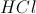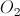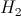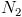Explanation:

Diatomic nitrogen,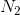, contains a triple bond between the two nitrogen atoms. Since there are six electrons being shared, the bond is stronger and the atoms are being pulled closer together. Diatomic oxygen,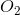,  has a double bond, which is not as strong. Diatomic hydrogen,, has a single bond, which have a bond length greater than either a double or triple bond. Hydrochloric acid,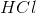, is an ionic compound and will have a bond length comparable to a single covalent bond.

### Example Question #1 : Other Bonding Concepts

What types of bonds are found in a single molecule of the following compound?

Ag(NH3)3

I. Covalent

II Hydrogen

III. Ionic

I only

II, III

III only

I, III

I, II

I, III

Explanation:

Ag(NH3)3 has covalent bonds (N-H) and ionic bonds (Ag-N).

A hydrogen bond is an intermolecular force between a hydrogen atom and an electronegative atom (O, N, F) that are in close proximity. Hydrogen bonds can occur within a given molecule (intramolecular), but more commonly occur between adjacent molecules (intermolecular). A solution of Ag(NH3)3 would show hydrogen bonding between the hydrogen and nitrogen of nearby molecules. The compound would not show intramolecular hydrogen. Since the question asks only for intramolecular interactions (single molecule), hydrogen bonding cannot be an answer choice.

### Example Question #36 : Bonding And Forces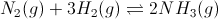Figure 1: Ammonia gas formation and equilibrium

Oxygen, hydrogen, and nitrgoen form diatomic molecules in their natural states. What is the order of these molecules by bond length?

O2 < H2 < N2

N2 < O2 < H2

H2 < O2 < N2

N< H2 < O2

O2 < N2 < H2

N2 < O2 < H2

Explanation:

We know from Lewis structures that diatomic hydrogen will have a single bond, diatomic oxygen will have a double bond, and diatomic nitrogen will have a triple bond. Triple bonds are stronger than double or single bonds, and the stronger the bond, the shorter the bond length. Nitrogen will have the shortest bond length.

We also know that the longest bond will be weakest. This mean hydrogen, with its single bond, would have the longest bond length. The answer should be N2 < O2 < H2.

### Example Question #1 : Other Bonding Concepts

Which of the following functional groups contains only central atoms with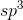hybridization?

Ester

Ketone

Aldehyde

Alcohol

Carboxylic acid

Alcohol

Explanation:

Out of the five given functional groups, only alcohols do not contain a central atom which is double bonded (meaning those atoms have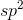hybridization). The only central atom of an alcohol, the oxygen atom in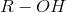, has single bonds to the R-group and a single hydrogen along with two lone pairs, giving ithybridization.

### Example Question #2 : Other Bonding Concepts

Which of the following types of organic compounds has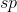hybridization?

Alkyne

Alkane

Ketone

Alkene

Carboxylic acid

Alkyne

Explanation:

The alkyne, a hydrocarbon containing a triple bond, has one sigma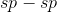bond, and two pi bonds, making a rod-like molecule with a bond angle of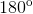andhybridization. It is the only type of compound of the choices to have a triple bond, making it the only possibility.

### Example Question #39 : Bonding And Forces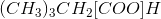What is the bond angle and molecular geometry formed by the atoms in brackets in the molecular formula above?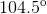; bent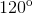; tetrahedral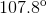; trigonal pyramidal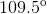; tetrahedral; trigonal planar; trigonal planar

Explanation:

The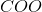group indicated by the question consists of a carbon atom bonded to two oxygen atoms (one with a double bond, and one with a single bond). The carbon is also bonded to the rest of the hydrocarbon chain by a single sigma bond. Because this carbon atom only has three electron domains and has no lone pairs, trigonal planar is the molecular geometry that best describes this piece of the larger molecule. The atoms bonded to this carbon molecule sit atfrom each other, an even division of the single plane in which these atoms sit.

### Example Question #581 : Ap Chemistry

What is the hybridization state of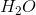?

sp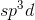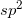has two sigma bonds between oxygen and hydrogen. It also has two pair of lone electrons on oxygen. The number of bonds and lone pairs contribute to the hybridization. For example, if a molecule consists of one sigma bond and one electron pair, the hybridization would be sp. If the molecule consists of two sigma bonds and one electron pair, the hybridization would be, and so forth.